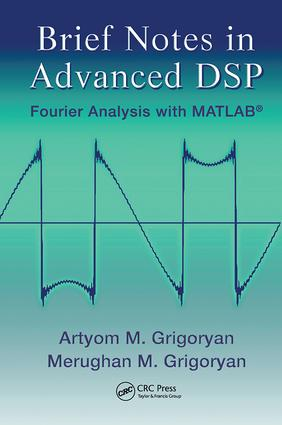# Brief Notes in Advanced DSP

## Fourier Analysis with MATLAB, 1st Edition

CRC Press

354 pages | 17 Color Illus. | 205 B/W Illus.

Paperback: 9781138117747
pub: 2017-11-15
\$82.95
x
Hardback: 9781439801376
pub: 2009-02-20
\$220.00
x
eBook (VitalSource) : 9781315218458
pub: 2018-10-03
from \$39.98

FREE Standard Shipping!

### Description

Based on the authors’ research in Fourier analysis, Brief Notes in Advanced DSP: Fourier Analysis with MATLAB® addresses many concepts and applications of digital signal processing (DSP). The included MATLAB® codes illustrate how to apply the ideas in practice.

The book begins with the basic concept of the discrete Fourier transformation and its properties. It then describes lifting schemes, integer transformations, the discrete cosine transform, and the paired transform method for calculating the discrete Hadamard transform. The text also examines the decomposition of the 1D signal by so-called section basis signals as well as new forms of 2D signal/image representation and decomposition by direction signals/images. Focusing on Fourier transform wavelets and Givens–Haar transforms, the last chapter discusses the problem of signal multiresolution.

This book presents numerous interesting problems and concepts of unitary transformations, such as the Fourier, Hadamard, Hartley, Haar, paired, cosine, and new signal-induced transformations. It aids readers in using new forms and methods of signals and images in the frequency and frequency-and-time domains.

Discrete Fourier Transform

Properties of the discrete Fourier transform

Fourier transform splitting

Fast Fourier transform

Codes for the paired FFT

Paired and Haar transforms

Integer Fourier Transform

Reversible integer Fourier transform

Lifting schemes for DFT

One-point integer transform

DFT in vector form

Roots of the unit

Codes for the block DFT

General elliptic Fourier transforms

Cosine Transform

Partitioning the DCT

Paired algorithm for the N-point DCT

Codes for the paired transform

Reversible integer DCT

Method of nonlinear equations

Canonical representation of the integer DCT

Generalized bit and transformations

Mixed Fourier transformations

Mixed transformations: continuous case

Paired Transform-Based Decomposition

Decomposition of 1D signals

2D paired representation

Fourier Transform and Multiresolution

Fourier transform

Representation by frequency-time wavelets

Time-frequency correlation analysis

Givens–Haar transformations

References

Index